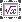On-line GuidesAll GuideseBook StoreiOS / AndroidLinux for BeginnersOffice ProductivityLinux InstallationLinux SecurityLinux UtilitiesLinux VirtualizationLinux KernelSystem/Network AdminProgrammingScripting LanguagesDevelopment ToolsWeb DevelopmentGUI Toolkits/DesktopDatabasesMail SystemsopenSolarisEclipse DocumentationTechotopia.comVirtuatopia.comAnswertopia.comHow To GuidesVirtualizationGeneral System AdminLinux SecurityLinux FilesystemsWeb ServersGraphics & DesktopPC HardwareWindowsProblem SolutionsPrivacy Policy## Formulas

KWord has the ability to directly create formulas using the formula editor common to all KOffice applications.

### Note

This is a formula editor for creating graphical versions of formulas. KWord does not currently have the ability to solve mathematical equations.

There are three ways to insert a formula into a document:

• By selecting Insert -> Formula from the menubar

• Using the keyboard shortcut: F4

• or by clickingon the toolbar.

KWord creates a formula frame at the current cursor location.

### Note

Formula frames behave differently than most other frames in KWord:

• All formula frames are inserted inline by default. This frame can later be converted to a non-inline frame later.

• Formula frames expand and contract depending on the resulting size of the formula.

• Formula frames automatically determine where in the line they are lined up.

### Moving a formula

By default, formula frames are created as inline frames by default. The formula frame will move with the text as any other inline frame would.

If you want to have more control over where the formula is placed, you can convert it to a framed formula by clicking on the frame surrounding the formula with the mouse button. A popup menu will appear. Simply select Inline Frame and the formula will now be a freely movable frame.

Once converted to a freely movable frame, you can move the frame just like any other frame in KWord.

### Removing a formula

To delete a formula in KWord, simply delete the frame around it.

### Editing a formula

Editing a formula is beyond the scope of this documentation. Please see the KFormula handbook for help with the editing of the formula.Published under the terms of the GNU General Public License Design by Interspire